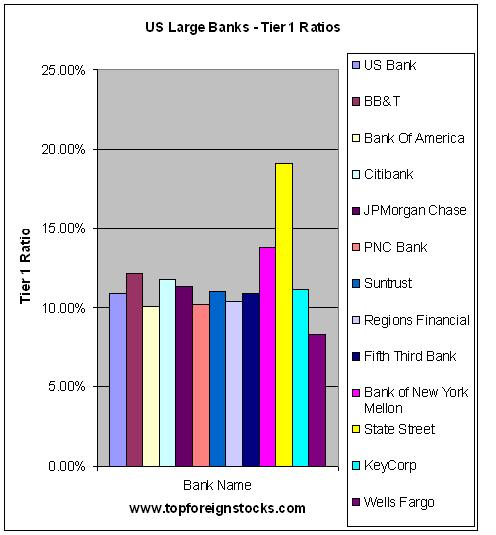# A Review of Tier 1 Capital Ratios of Large US Banks

A bank’s Tier 1 Capital Ratio is an indicator of its strength and ability to absorb losses. RegulatorsÂ  in the USA and other countries are now using this factor in addition to others to identify banks that are likely to collapse.

The Tier 1 capital ratio is the ratio of a bank’s core equity capital to its total risk-weighted assets (Source: Wikipedia). This ratio is considered to be a more reliable measure of financial strength than other numbers that can be calculated to evaluate a bank. In general, the higher the ratio the better the bank. For example, a bank having a Tier 1 ratio of 20% is better than the one with less than 10%. Higher Tier 1 ratio implies that the bank is being run very conservatively.

The following chart shows the Tier 1 Ratios of thirteen largeÂ  banks in USA.These numbers were pulled from their first quarter, 2009 earnings report. The Tier1 ratios include TARP funds if a bank accepted it. Hence in some cases the Tier 1 Ratio is higher than it was originally. Some of these banks listed in the chart below repaid billions in TARP funds this week.

Large US Banks Tier 1 Capital RatiosÂ

ChartTable

[TABLE=155]

Source: Bank 1Q,2009 Earning Reports

State Street (STT) is highly conservative since it has a Tier 1 ratio of 19.13%. Wells Fargo(WFC) on the other hand had the lowest ratio at 8.28%. Despite heavy TARP infusions Bank of America (BAC) and Citibank (C) have Tier 1 ratios of just 10.09% and 11.80% respectively. Other than State Street, Bank of New York Mellon (BK) and BB&T (BBT) have high Tier 1 ratios of above 12%. Some might argue that the capital ratios of US banks that accepted government funds may not be a good indicator of financial strength since the numbers are inflated. However it is still worth monitoring on a quarterly basis as wild swings in the Tier 1 ratio can be indicator of other problems.

1.Ron Gruendl
2.David